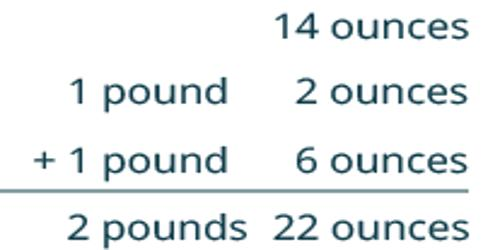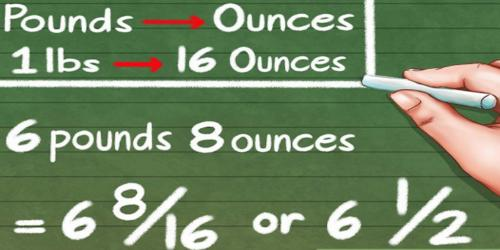Mathematic

# How to Add Pounds and Ounces?If the number of ounces is greater than 16, divide the ounces by 16. The quotient is the number of pounds that need to be added to the other pounds. The remainder is the number of extra ounces.

Add the original pounds plus the whole pounds resulting from the addition of the ounces.

Since there are 16 ounces in a pound, this is how to add pounds and ounces:

If the number of ounces is 16 or more, regroup the ounces into pounds: Divide the ounces by 16.

Add the quotient to the number of pounds.

The remainder is the number of extra ounces.

If the subtracted number of ounces is greater than the original number of ounces, you can “borrow” 16 ounces from the original number of pounds: Reduce the original number of pounds by 1. Increase the original number of ounces by 16.Example: Add 5 pounds 8 ounces plus 3 pounds 10 ounces

Add 8 ounces to 10 ounces:

8 + 10 = 18 ounces

The number of ounces is greater than 16 so divide by 16:

18 ÷ 16 = 1 pound 2 ounces